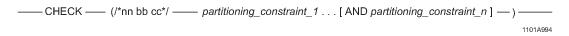# 15.00 - Partitioning CHECK Constraint for Partitioned Tables With 8-Byte Partitioning - Teradata Database

## Teradata Database SQL Data Definition Language Detailed Topics

Product
Release Number
15.00
Content Type
Programming Reference
Publication ID
B035-1184-015K
Language
English (United States)

### Partitioning CHECK Constraint for Partitioned Tables With 8‑Byte Partitioning

Just as it does for a 2-byte partitioned primary index, Teradata Database derives a partitioning CHECK constraint for 8‑byte partitioning from the partitioning expressions.

The text for this CHECK constraint that is derived for table constraints, partitioning constraints, and named constraints, cannot exceed 16,000 characters. Otherwise, the system returns an error to the requestor.

Teradata Database uses this form of partitioning CHECK constraint when any one of the following things is true about the partitioning expression for a table or join index.

• A partitioning expression for at least 1 level is defined only by a RANGE_N function with a BIGINT data type.
• At least 1 partitioning level in the partitioning expression specifies an ADD clause.
• The table or join index has 8-byte partitioning.
• The table or join index is column-partitioned.
• The number of partitions for at least 1 partitioning level other than level 1 of a populated table changes when the table is altered with an ALTER TABLE request.
• The number of partitions for at least 1 partitioning level other than level 1 of an unpopulated table decreases when the table is altered with an ALTER TABLE request.
• The cost profile constant PartitioningConstraintForm is set to 1.
• The format for this partitioning CHECK constraint text for multilevel partitioning with 8‑byte partitioning, which applies to both row‑partitioned and column‑partitioned tables and join indexes, is as follows.where n is the number of the highest partitioning level defined for the partitioning expression. For example, if the partitioning expression for a table has 18 partitioning levels, then the value for n is 18, and the partitioning CHECK constraint documents 18 partitioning expressions.

Each of the individual partitioning constraints in the partitioning CHECK constraint corresponds to a level of partitioning in the order defined for the table.

where:

 Syntax element … Specifies … nn the number of partitioning levels. For 2‑byte partitioning, nn ranges between 01 and 15, inclusive. For 8‑byte partitioning, nn ranges between 01 and 62, inclusive. bb the number of bytes used to store the internal partition number in the row header. For 2‑byte partitioning, bb = 02. For 8‑byte partitioning, bb = 08. cc the column partitioning level for a column‑partitioned table. If the table is not column‑partitioned, cc = 00. If the table is column‑partitioned, the value of cc ranges between 01 and nn, inclusive, where nn is the number of partitioning levels for the table. partitioning_constraint_i the partitioning expression at partitioning level i. If the table or join index is row‑partitioned at partitioning level i, the form of partitioning_constraint_i is as follows. `partitioning_expression_i /*i d+a*/ IS NOT NULL` If the table or join index is column‑partitioned at partitioning level i, the form of partitioning_constraint_i is as follows. `PARTITION#Li /*i d+a*/=1` where: partitioning_expression_i is the partitioning expression at level i. i is the partitioning level of the partitioning expression for the table or join index. The value ranges between 1 and nn, inclusive. Teradata Database does not store leading zeros for the value of i. d is the number of defined partitions for partitioning level i. For a column partitioning level, this value includes the 2 column partitions reserved for internal use. Teradata Database does not store leading zeros for the value of d. a is either the number of partitions that could be added to the partitioning level (which might be 0) or X. X occurs for levels 2 and higher if this partitioning CHECK constraint form is only being used because the cost profile constant PartitioningConstraintForm is set to 1 to force use of the this partitioning CHECK constraint form in all cases. If Teradata Database would use this form of partitioning CHECK constraint regardless of the setting of PartitioningConstraintForm, or if the partitioning level is level 1, the value of a is an INTEGER number. Teradata Database does not store leading zeros for the value of a.

See “ALTER TABLE (Basic Table Parameters)” on page 31 for more information about the ALTER TABLE statement.

The following example demonstrates the form of partitioning CHECK constraint that Teradata Database uses for 8‑byte partitioning. Assume you create the following table.

` `
`     CREATE TABLE orders (`
`       o_orderkey      INTEGER NOT NULL,`
`       o_custkey       INTEGER,`
`       o_orderstatus   CHARACTER(1) CASESPECIFIC,`
`       o_totalprice    DECIMAL(13,2) NOT NULL,`
`       o_orderdate     DATE FORMAT 'yyyy-mm-dd' NOT NULL,`
`       o_comment       VARCHAR(79))`
`     NO PRIMARY INDEX`
`     PARTITION BY (RANGE_N(o_custkey BETWEEN  0`
`                                     AND 100000`
`                                     EACH     1),`
`                   COLUMN)`
`     UNIQUE INDEX (o_orderkey);`
` `

The product of the maximum partition number of each partitioning level is 100,000 (100,000 * 1), which is greater than 65,535, so the table has 8‑byte partitioning. The table‑level partitioning CHECK constraint text for this 8‑byte partitioning is as follows.

`     CHECK (/*02 08 02*/ RANGE_N(o_custkey BETWEEN  0 `
`                                           AND 100000`
`                                           EACH     1`
`            /*1 100001+485440633518572409*/`
`            IS NOT NULL AND PARTITION#L2 /*2 9+10*/=1)`
` `

You can use the following request to retrieve the level for the column partitioning for each of the objects that have column partitioning in the system.

` `
`     SELECT DBaseId, TVMId, ColumnPartitioningLevel `
`            (TITLE ‘Column Partitioning Level’)`
`     FROM DBC.TableConstraints`
`     WHERE ConstraintType = 'Q' `
`     AND   ColumnPartitioningLevel >= 1`
`     ORDER BY DBaseId, TVMId;`

See “Rules and Usage Notes for Partitioned Tables” on page 625 and “Restrictions for Multilevel Partitioning” on page 634 for information about the table‑level partitioning CHECK constraints that Teradata Database creates for single‑level and multilevel partitioning tables and join indexes with 2‑byte partitioning.